# Jest 零基础入门# 三、学习前提

• js、es6 基础语法
• node、npm 相关知识
• git 的相关操作
• react或者vue，至少了解一个
• 状态管理工具，至少了解一个

# 四、背景及原理

``````// math.js

return a + b;
}

function minus(a, b) {
return a - b;
}

``````const result = add(3, 7);
const expect = 10;

if (result !== expect) {
throw new Error(`3 + 7 应该等于\${expect}，结果却是\${result}`);
}

``````const result = minus(3, 3);
const expect = 0;

if (result !== expect) {
throw new Error(`3 - 3 应该等于\${expect}，结果却是\${result}`);
}

``````// 测试 3 + 3 是否等于 6

// 测试 3 - 3 是否等于 0
expect(minus(3, 3)).toBe(0);

`expect 方法`实现：

``````function expect(result) {
return {
toBe(actual) {
if (result !== actual) {
throw new Error("预期值和实际值不相等");
}
},
};
}

``````test("测试加法 3 + 3", () => {
});

test("测试减法 3 - 3", () => {
expect(minus(3, 3)).toBe(0);
});

`test 方法`实现：

``````function test(desc, fn) {
try {
fn();
console.log(`\${desc} 通过测试`);
} catch {
console.log(`\${desc} 没有通过测试`);
}
}

# 五、Jest 框架入门

## 5.1 Jest 框架介绍

• 性能好
• 功能齐全
• 易用性很好

`Jest` 在这三个方面做的非常好（当然了，像`Jasmine``mocha`这些主流前端测试框架做的也不错）。

• 速度快（可以自动监测修改过的代码，不会重复测试）
• API 简单、数量少
• 易配置
• 隔离性好
• 监控模式
• IDE 整合（比如vs code）
• Snapshot（快照测试）
• 多项目并行
• 覆盖率
• Mock 丰富
• 对新技术支持度好

## 5.2 使用 Jest 修改自动化测试样例

`npm init`

``````npm install jest -D

（注：-D 是 --save -dev 的缩写）

``````// math.js

return a + b;
}

function minus(a, b) {
return a - b;
}

function multi(a, b) {
return a * b;
}

module.exports = { add, minus, multi };

``````// math.test.js

const { add, minus, multi } = require("./math");

test("测试加法 3 + 3", () => {
});

test("测试减法 3 - 3", () => {
expect(minus(3, 3)).toBe(0);
});

test("测试乘法 3 * 3", () => {
expect(multi(3, 3)).toBe(9);
});

``````"scripts": {
"test": "jest"
},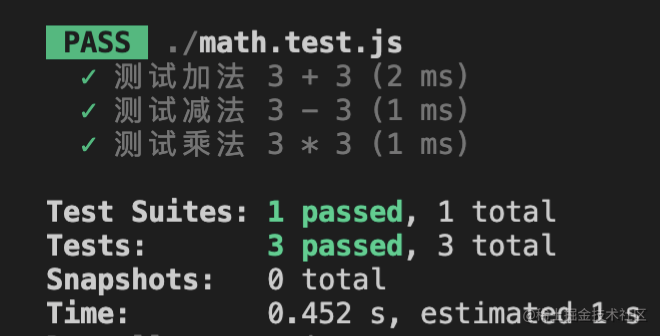``````// math.test.js

const { add, minus, multi } = require("./math");

test("测试加法 3 + 3", () => {
});

test("测试减法 3 - 3", () => {
expect(minus(3, 3)).toBe(0);
});

test("测试乘法 3 * 3", () => {
expect(multi(3, 3)).toBe(10); // 错误的结果
});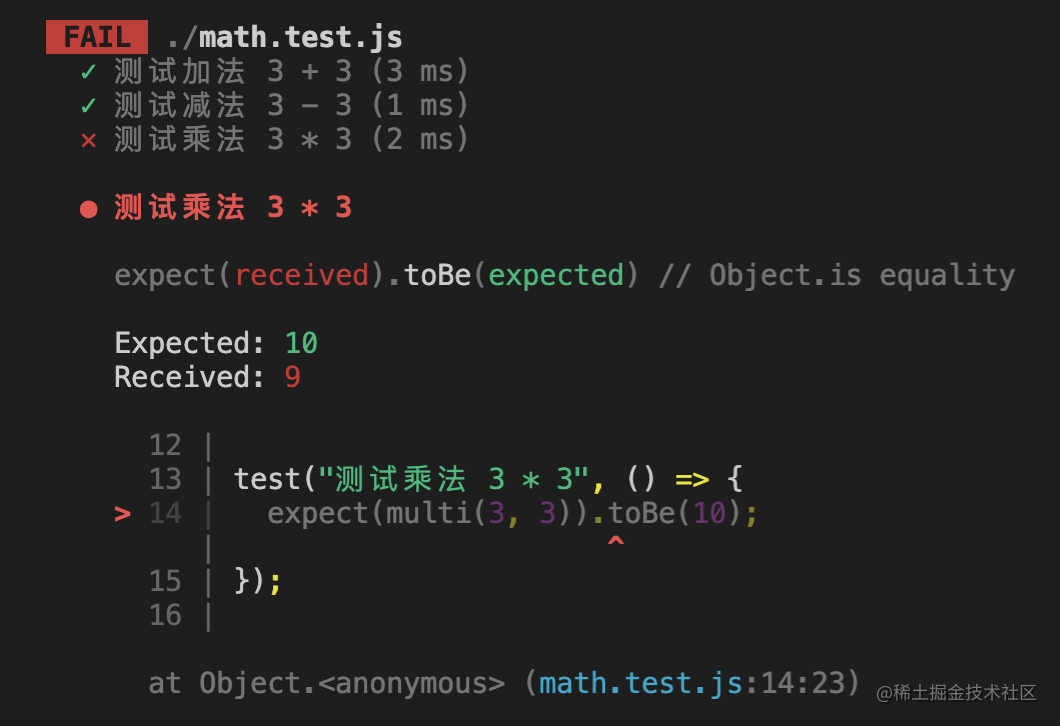## 5.3 Jest 的简单配置

`Jest``Webpack` 一样都有`默认配置`，我们可以运行 `npx jest --init` 命令来 初始化默认配置。在运行命令行的时候，会弹出一些选项，比如：

• 是否需要开启`typescript`
• 是否需要生成`覆盖率报告`
• 选择`node 环境`或者`浏览器环境`
• 在测试完成之后，是否需要进行一些`清除工作`
• ……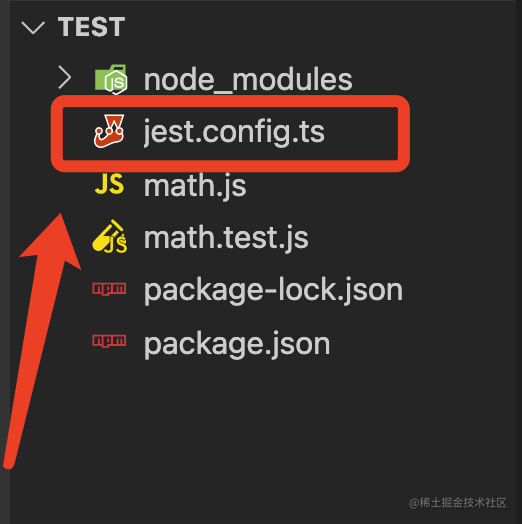### 1. 代码测试覆盖率：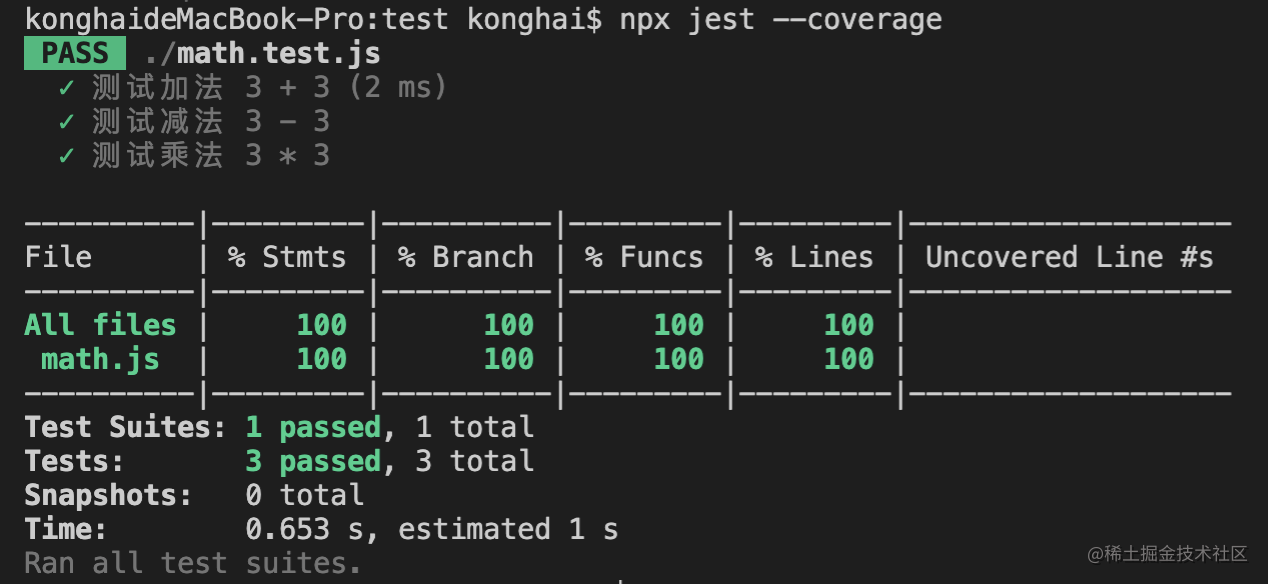``````"scripts": {
"test": "jest",
"coverage": "jest --coverage"
},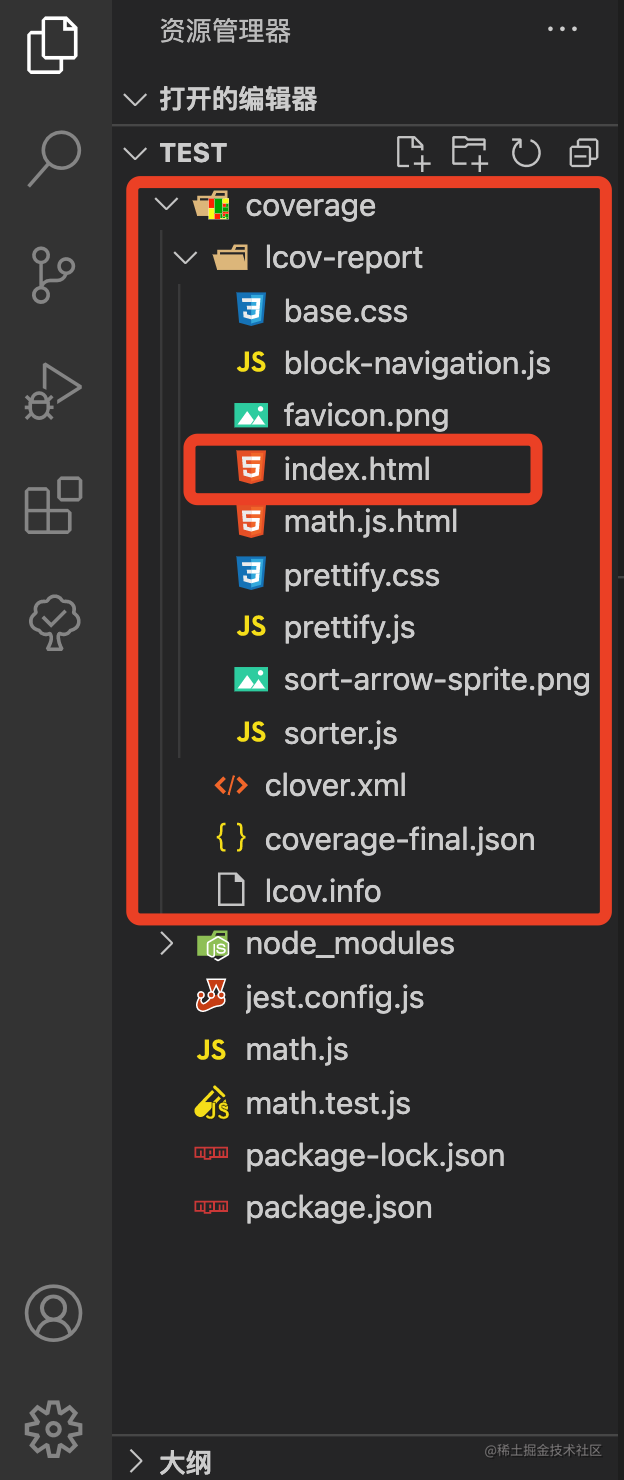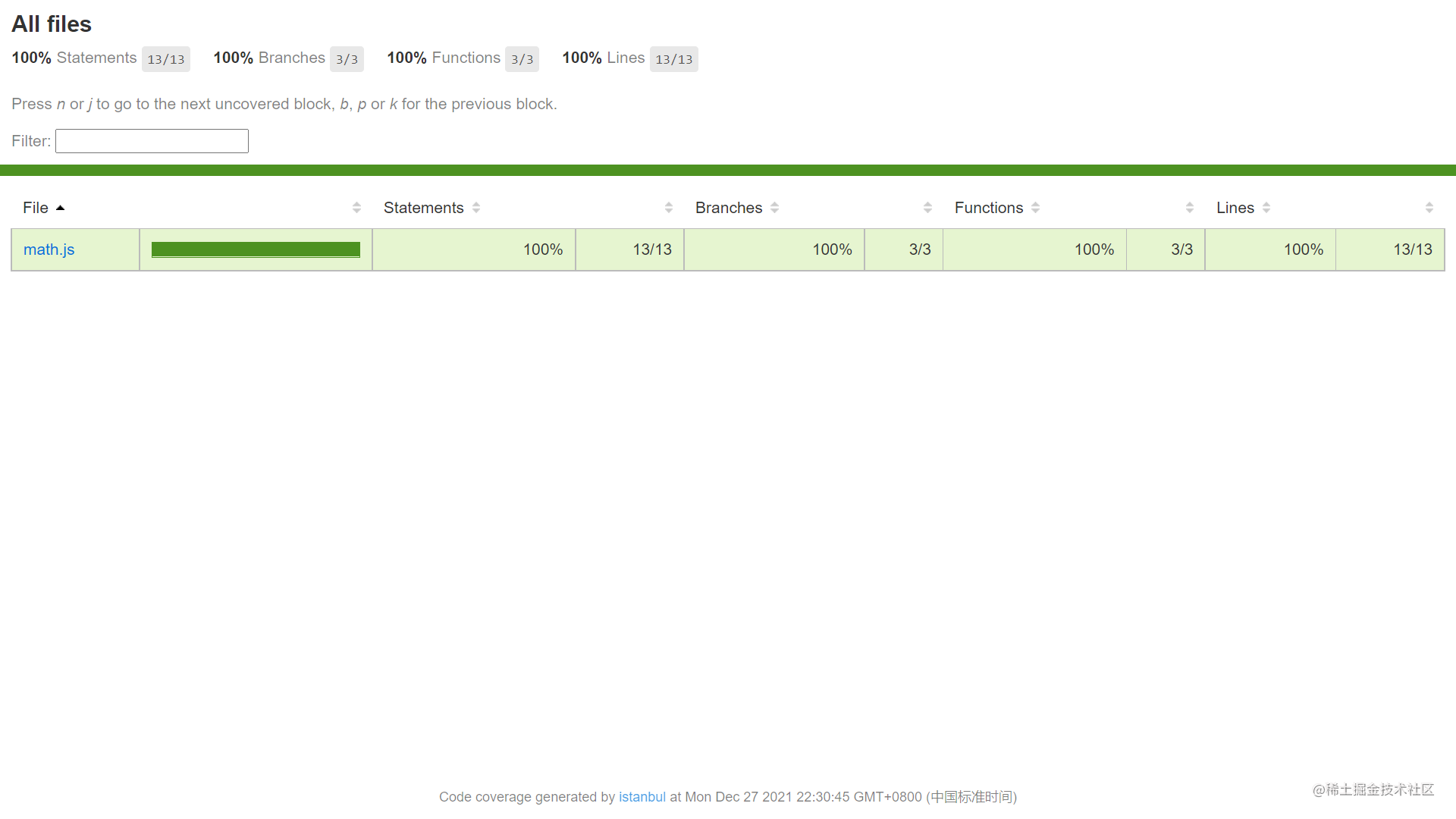tips: 配置文件中的`coverageDirectory`就是代码测试覆盖率生成报告`所在的文件夹`的名字：

``````module.exports = {
coverageDirectory: "abc"
};

### 2. 把 commonjs 改成 es module

``````// math.js

return a + b;
}

export function minus(a, b) {
return a - b;
}

export function multi(a, b) {
return a * b;
}

``````// math.test.js

import { add, minus, multi } from "./math";

test("测试加法 3 + 3", () => {
});

test("测试减法 3 - 3", () => {
expect(minus(3, 3)).toBe(0);
});

test("测试乘法 3 * 3", () => {
expect(multi(3, 3)).toBe(9);
});

• `@babel/core`：babel核心库
• `@babel/preset-env`：进行 ES 语法转换的库
• `babel-jest`：和 Jest 通信的库，用来检测是否安装了上面两个依赖

``````npm install @babel/core @babel/preset-env -D

（注：-D 是 --save -dev 的缩写）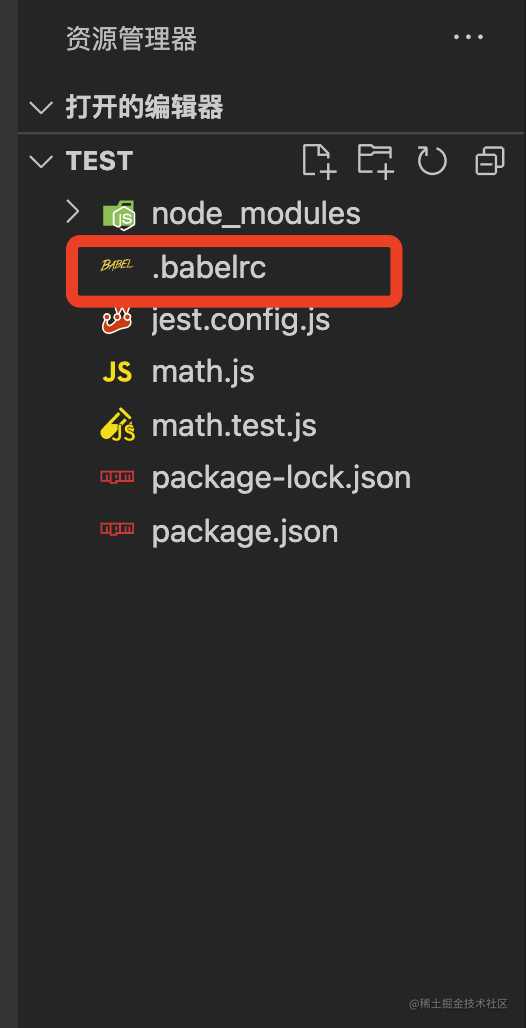``````{
"presets": [["@babel/preset-env", { "targets": { "node": "current" } }]]
}

## 5.4 Jest 中的匹配器（matchers）

``````expect(1 + 1).toBe(2);

• `toBe`：测试两个对象的值是否相等，类似于 js 中的 === 运算符
``````// toBe

test("测试加法交换律", () => {
for (let x = 0; x <= 10; x++) {
for (let y = 0; y <= 10; y++) {
expect(x + y).toBe(y + x);
}
}
});

• `toEqual`：测试两个对象的原始值是否相等，只检查内容，不检查引用
``````// toEqual

const can1 = { value: "hello" };
const can2 = { value: "hello" };

test("测试 can1 和 can2 的内容是否相等", () => {
expect(can1).toEqual(can2);
});

• `toBeNull`：测试对象的值是否为null，效果相当于`.toBe(null)`
``````// toBeNull

const value = null;

test("测试值是否为 null", () => {
expect(value).toBeNull();
});

• `toBeUndefined`：测试对象的值是否为undefined，效果相当于`.toBe(undefined)`
``````// toBeUndefined

const value = undefined;

test("测试值是否为 undefined", () => {
expect(value).toBeUndefined();
});

• `toBeDefined`：测试值是否被定义过，除了undefined之外都会通过测试
``````// toBeDefined

const value = 1;

test("测试值是否被定义过", () => {
expect(value).toBeDefined();
});

• `toBeTruthy`：检查值转成布尔值之后是否为真值
• `toBeFalsy`：检查值转成布尔值之后是否为假值
``````// toBeTruthy、toBeFalsy

test("测试是否为真值", () => {
expect(0).toBeTruthy(); // 不通过
expect("").toBeTruthy(); // 不通过
expect(null).toBeTruthy(); // 不通过
expect(false).toBeTruthy(); // 不通过
expect(undefined).toBeTruthy(); // 不通过
});

test("测试是否为假值", () => {
expect(0).toBeFalsy(); // 通过
expect("").toBeFalsy(); // 通过
expect(null).toBeFalsy(); // 通过
expect(false).toBeFalsy(); // 通过
expect(undefined).toBeFalsy(); // 通过
});

• `not`：取反匹配器，相当于 js 中的 ! 运算符
``````// not

test("测试值是否不为 aaa", () => {
expect("hello").not.toBe("aaa");
});

test("测试值是否不为 null", () => {
expect([]).not.toBeNull();
});

test("测试值是否不为 undefined", () => {
expect({}).not.toBeUndefined();
});

• `toBeGreaterThan`：检查接收值是否大于期待值
``````// toBeGreaterThan

test("测试 10 是否大于 9", () => {
expect(10).toBeGreaterThan(9);
});

• `toBeLessThan`：检查接收值是否小于期待值
``````// toBeLessThan

test("测试 10 是否小于 20", () => {
expect(10).toBeLessThan(20);
});

• `toBeGreaterThanOrEqual`：检查接收值是否大于等于期待值
``````// toBeGreaterThanOrEqual

test("测试 10 是否大于等于 10", () => {
expect(10).toBeGreaterThanOrEqual(10);
});

• `toBeLessThanOrEqual`：检查接收值是否小于等于期待值
``````// toBeLessThanOrEqual

test("测试 10 是否小于等于 10", () => {
expect(10).toBeLessThanOrEqual(10);
});

• `toBeCloseTo`：检查浮点数是否接近（是否近似相等）
``````// toBeCloseTo

test("测试 0.1 + 0.2 是否等于 0.3", () => {
expect(0.1 + 0.2).toBe(0.3); // 不通过
expect(0.1 + 0.2).toBeCloseTo(0.3); //通过
});

• `toMatch`：检查值是否和字符串或者正则相匹配
``````// toMatch

test("测试字符串是否包含 baidu", () => {
expect("www.baidu.com").toMatch("baidu");
expect("www.baidu.com").toMatch(/baidu/);
});

• `toContain`：检查数组中是否包含某一项（类似于 js 中的 includes 方法）
``````// toContain

test("测试 list 中是否包含 3", () => {
const list = [1, 2, 3];
expect(list).toContain(3);
});

• `toThrow`：测试函数在调用时是否有异常抛出
``````// toThrow

const fn1 = () => {
console.log("hello");
};

const fn2 = () => {
throw new Error("this is a new err");
};

test("测试 fn1、fn2 调用时是否有异常", () => {
expect(fn1).toThrow(); // 不通过
expect(fn2).toThrow(); // 通过
});

`Jest` 中，除了上面这些列举出来的常用的`匹配器`之外，还有很多匹配器为我们提供。不需要记住所有，知道常用的几个即可。如果想了解更多，可以点击这里查看官方文档

## 5.5 Jest 命令行工具的使用

``````"scripts": {
"test": "jest --watchAll",
"coverage": "jest --coverage"
},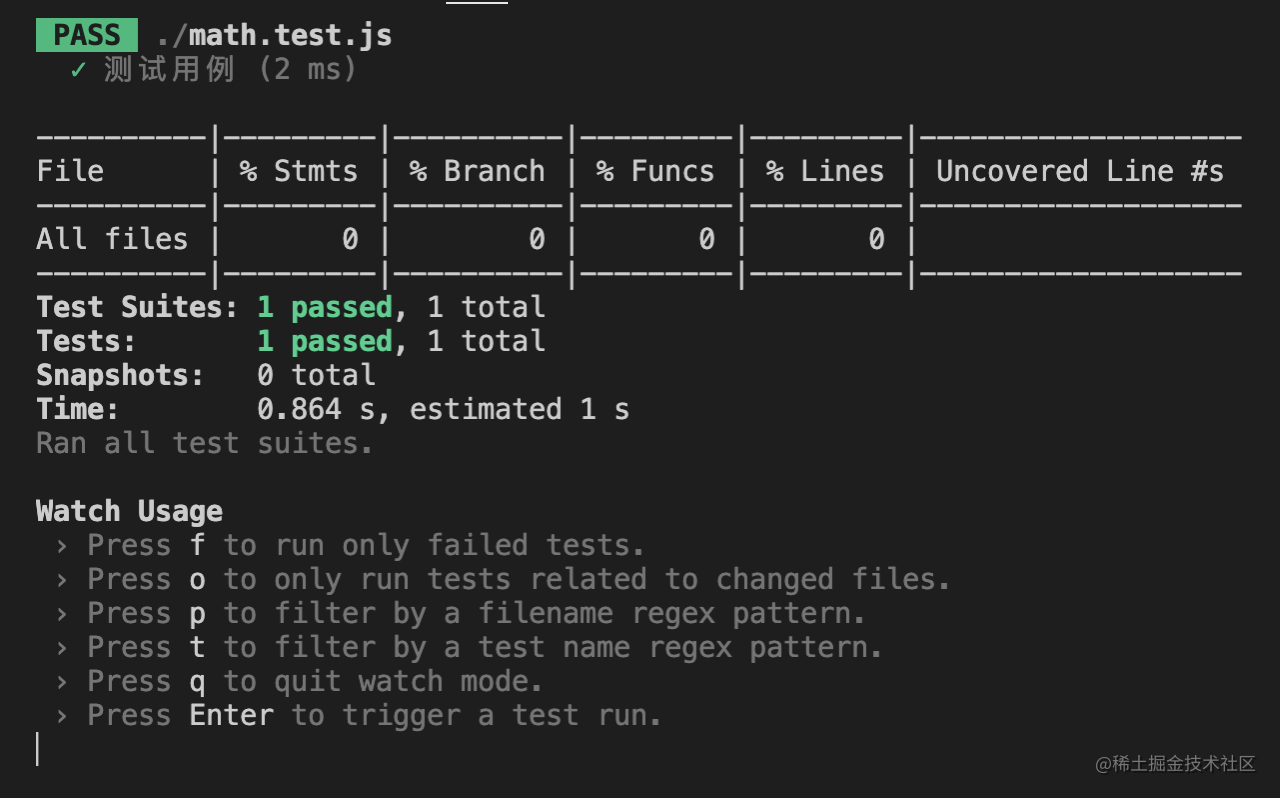• `f`键：只运行失败的测试用例
• `o`键：只运行发生更改的测试用例
• `p`键：按文件名regex模式进行筛选
• `t`键：按测试名称regex模式进行筛选
• `q`键：退出监测模式
• `Enter`键：触发测试用例

`Jest` 中有很多`命令行工具`，合理的使用命令行，会让我们的测试变的更加灵活、好用。

## 5.6 异步代码的测试

``````npm install axios --save

``````{
"success": true
}

``````import axios from "axios";

export const getData = () => {
return axios.get("http://www.dell-lee.com/react/api/demo.json");
};

### 方法一：Promises 规范

#### 测试成功的返回值：

``````import { getData } from "./index";

test("测试 getData 的返回值为 { success: true }", () => {
return getData().then(res => {
expect(res.data).toEqual({ success: true });
});
});

#### 测试失败的返回值：

``````import { getData } from "./index";

test("测试 getData 的返回值包含 404", () => {
return getData().catch(err => {
expect.assertions(1);
expect(err.toString()).toMatch("404");
});
});

### 方法二：.resolves 匹配器 / .rejects 匹配器

#### 测试成功的返回值：

``````import { getData } from "./index";

test("测试 getData 的返回值为 { success: true }", () => {
return expect(getData()).resolves.toEqual({ success: true });
});

#### 测试失败的返回值：

``````import { getData } from "./index";

test("测试 getData 的返回值包含 404", () => {
return expect(getData()).rejects.toMatch("404");
});

### 方法三：Async / Await

#### 测试成功的返回值：

``````import { getData } from "./index";

test("测试 getData 的返回值为 { success: true }", async () => {
const { data } = await getData();
expect(data).toEqual({ success: true });
});

#### 测试失败的返回值：

``````import { getData } from "./index";

test("测试 getData 的返回值包含 404", async () => {
expect.assertions(1);
try {
await getData();
} catch (err) {
expect(err.message).toMatch("404");
}
});

### 方法四：Async、Await 和 .resolves、.rejects 结合使用

``````import { getData } from "./index";

test("测试 getData 返回成功", async () => {
await expect(getData()).resolves.toEqual({ success: true });
});

test("测试 getData 返回失败", async () => {
await expect(getData()).rejects.toMatch("404");
});

## 5.7 Jest 中的钩子函数

``````// index.js

class Counter {
constructor() {
this.number = 0;
}
this.number++;
}
minus() {
this.number--;
}
}

export default Counter;

• 在实例化的时候定义了`number`，初始值是`0`
• 定义了静态方法`add`，执行结果为`number``1`
• 定义了静态方法`minus`，执行结果为`number``1`

``````// index.test.js

import Counter from "./index";

const counter = new Counter();

test("测试 Counter 的 add 方法", () => {
expect(counter.number).toBe(0);
expect(counter.number).toBe(1);
});

``````// index.test.js

import Counter from "./index";

const counter = new Counter();

test("测试 Counter 的 add 方法", () => {
expect(counter.number).toBe(0);
expect(counter.number).toBe(1);
});

test("测试 Counter 的 minus 方法", () => {
expect(counter.number).toBe(0);
counter.minus();
expect(counter.number).toBe(-1);
});

• 测试 `add` 方法：通过
• 测试 `minus` 方法：不通过

``````// index.test.js

import Counter from "./index";

let counter = null;

beforeEach(() => {
counter = new Counter();
});

test("测试 counter 的 add 方法", () => {
expect(counter.number).toBe(0);
expect(counter.number).toBe(1);
});

test("测试 counter 的 minus 方法", () => {
expect(counter.number).toBe(0);
counter.minus();
expect(counter.number).toBe(-1);
});

`beforeEach()` 的作用是在每个测试用例执行之前执行里面的回调函数，如果你需要在测试开始之前对很多个测试做一些重复的工作，比如要初始化状态，你就可以使用它。

• `beforeAll`：在所有测试用例执行之前调用（调用一次）
• `afterAll`：在所有测试用例执行之后调用（调用一次）
• `beforeEach`：在每个测试用例执行之前调用（调用多次）
• `afterEach`：在每个测试用例执行之后调用（调用多次）

## 5.8 钩子函数的作用域

``````// index.test.js

describe("测试分组1", () => {
beforeAll(() => {
console.log("测试分组1 - beforeAll");
});
afterAll(() => {
console.log("测试分组1 - afterAll");
});
test("测试", () => {
console.log("测试分组1 测试");
expect(1 + 1).toBe(2);
});
});

describe("测试分组2", () => {
beforeAll(() => {
console.log("测试分组2 - beforeAll");
});
afterAll(() => {
console.log("测试分组2 - afterAll");
});
test("测试", () => {
console.log("测试分组2 测试");
expect(1 + 1).toBe(2);
});
});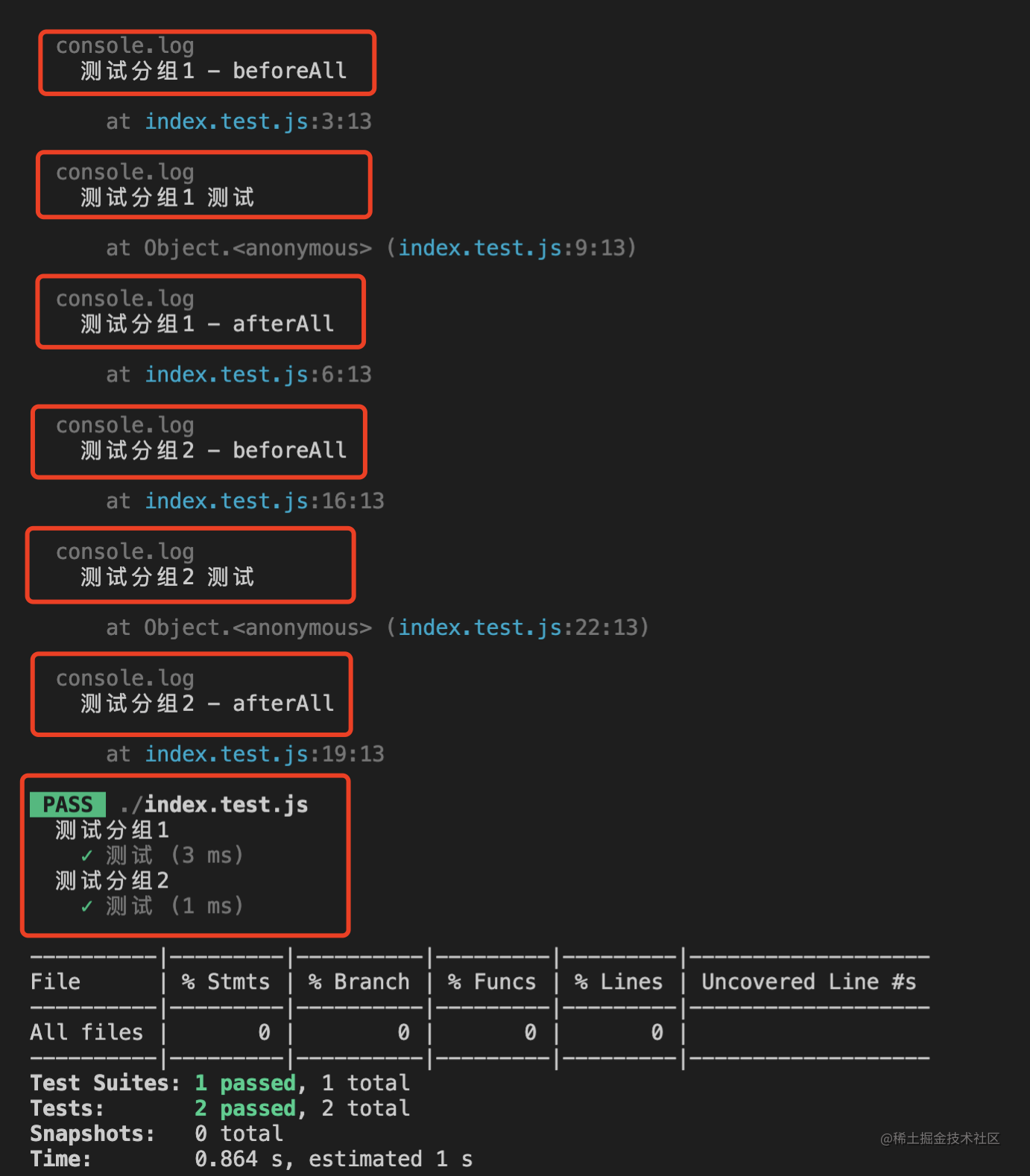• 如果我们不进行分组，相当于在最外面写了一层`describe`
• 除此之外，实际上，在`describe`里面还能嵌套`describe`，就像下面这样：
``````// index.test.js

describe("第一层", () => {
beforeAll(() => console.log("第一层 - beforeAll"));
describe("第二层", () => {
beforeAll(() => console.log("第二层 - beforeAll"));
describe("第三层", () => {
beforeAll(() => console.log("第三层 - beforeAll"));
test("测试", () => {
console.log("测试");
expect("hello" + " " + "world").toBe("hello world");
});
});
});
});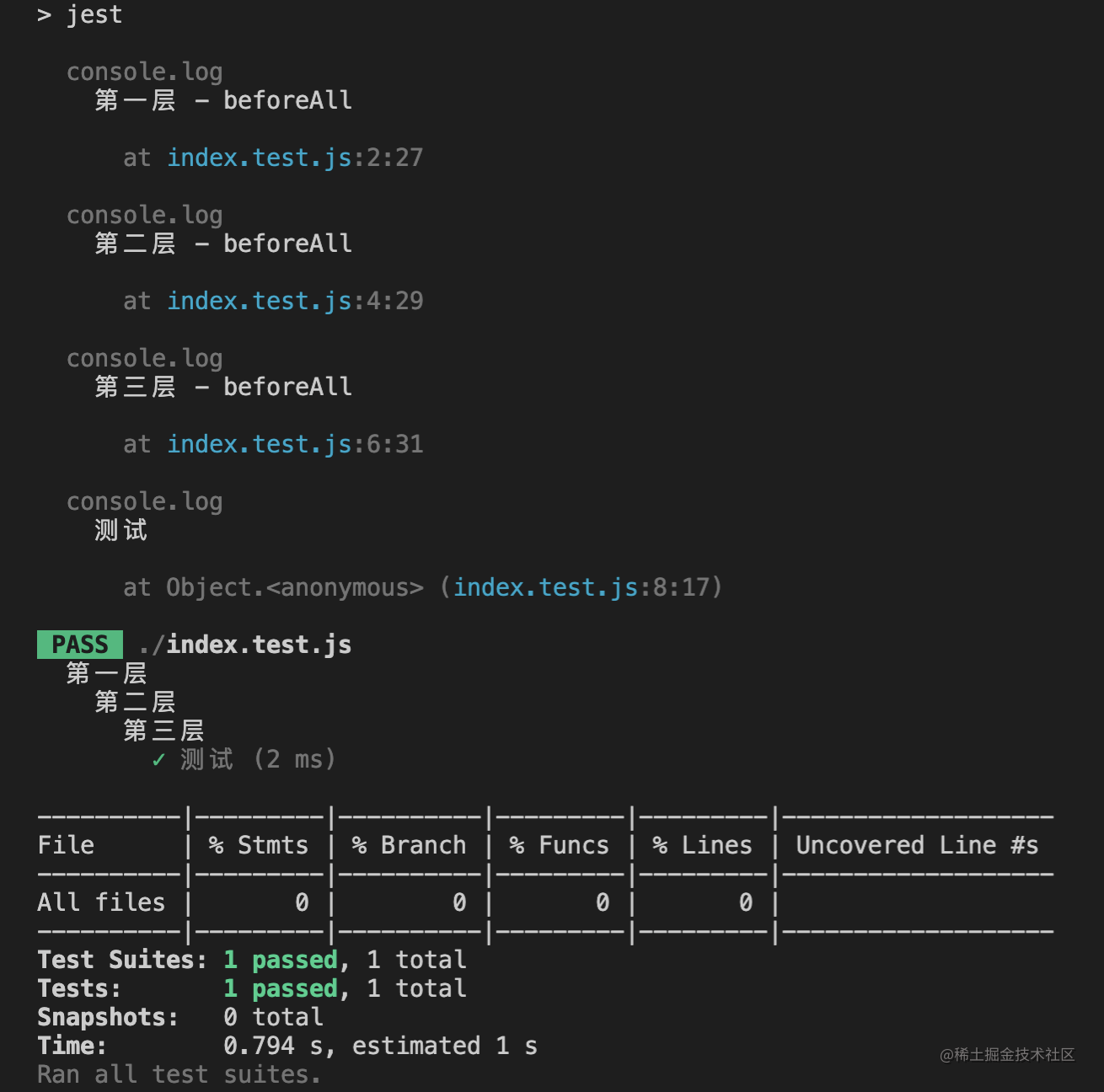• 每一个 `describe` 都可以有自己的`钩子函数`
• 每一个 `describe` 都有自己的`作用域`
• 每一个 `钩子函数`也有自己的作用域，就是当前所在的 `describe`
• 每一个 `describe` 里面的`钩子函数`对自己`作用域`下面所有的`测试用例`都生效
• 如果 `describe` 是多层嵌套的，那么测试用例执行的顺序是`由外到内`

``````// index.test.js

test.only("这个测试会被执行", () => {
expect("A").toBe("A");
});

test("这个测试会被跳过", () => {
expect("B").toBe("B");
});

## 5.9 Jest 中的 Mock

### 1. 函数的 Mock

`Mock` 函数提供的以下三种特性，在我们写测试代码时十分有用：

• 捕获函数调用情况
• 设置函数返回值
• 改变函数的内部实现

#### 1.1 测试函数是否被正常调用

``````// index.js

export const runCallback = callback => {
callback();
};

``````// index.test.js

import { runCallback } from "./index";

test("测试 runCallback", () => {
const func = jest.fn(); // 生成 mock 函数，捕获函数的调用
runCallback(func); // 调用 mock 函数
expect(func).toBeCalled(); // toBeCalled 匹配器用来检查函数是否被调用过
});

#### 1.2 测试函数调用次数是否正确

``````// index.js

export const runCallback = callback => {
callback();
};

``````// index.test.js

import { runCallback } from "./index";

test("测试调用次数", () => {
const func = jest.fn(); // 生成 mock 函数，捕获函数的调用
runCallback(func); // 第一次调用 mock 函数
runCallback(func); // 第二次调用 mock 函数
runCallback(func); // 第三次调用 mock 函数
expect(func.mock.calls.length).toBe(3); // 检查函数是否被调用了三次
});

#### 1.3 测试函数是否返回 undefined

``````// index.js

export const runCallback = callback => {
callback();
};

``````// index.test.js

import { runCallback } from "./index";

test("测试返回值", () => {
const func = jest.fn(); // 生成 mock 函数，捕获函数的调用
expect(runCallback(func)).toBeUndefined(); // 检查函数是否返回 undefined
});